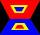# Right triangle + trapezoid - math problems

#### Number of problems found: 97

• Inner anglesThe magnitude of the internal angle at the main vertex C of the isosceles triangle ABC is 72°. The line p, parallel to the base of this triangle, divides the triangle into a trapezoid and a smaller triangle. How big are the inner angles of the trapezoid?
• Trapezoid ABCD v2Trapezoid ABCD has a length of bases in ratio 3:10. The area of triangle ACD is 825 dm2. What is the area of trapezoid ABCD?
• Isosceles trapezoidIn an isosceles trapezoid KLMN intersection of the diagonals is marked by the letter S. Calculate the area of trapezoid if /KS/: /SM/ = 2:1 and a triangle KSN is 14 cm2.
• Trapezoid MO-5-Z8Trapezoid KLMN has bases 12 and 4 cm long. The area of triangle KMN is 9 cm2. What is the area of the trapezoid KLMN?
• Rectangular trapezoidHow many inner right angles have a rectangular trapezoid?
• Trapezoid - diagonalA trapezoid has a length of diagonal AC crossed with diagonal BD in the ratio 2:1. The triangle created by points A, cross point of diagonals S and point D has area 164 cm2. What is the area of the trapezoid?
• Trapezoid 25Trapezoid PART with AR||PT has (angle P=x) and (angle A=2x) . In addition, PA = AR = RT = s. Find the length of the median of Trapezoid PART in terms of s.
• Trapezoid MO-5-Z8ABCD is a trapezoid that lime segment CE is divided into a triangle and parallelogram, as shown. Point F is the midpoint of CE, DF line passes through the center of the segment BE, and the area of the triangle CDE is 3 cm2. Determine the area of the trape
• The sides 2The sides of a trapezoid are in the ratio 2:5:8:5. The trapezoid’s area is 245. Find the height and the perimeter of the trapezoid.
• A trapezoidA trapezoid with a base length of a = 36.6 cm, with angles α = 60°, β = 48° and the height of the trapezoid is 20 cm. Calculate the lengths of the other sides of the trapezoid.
• Trapezoid thirdsThe ABCD trapezoid with the parallel sides of the AB and the CD and the E point of the AB side. The segment DE divides the trapezoid into two parts with the same area. Find the length of the AE line segment.
• MO Z9–I–2 - 2017In the VODY trapezoid, VO is a longer base and the diagonal intersection K divides the VD line in a 3:2 ratio. The area of the KOV triangle is 13.5 cm2. Find the area of the entire trapezoid.
• Isosceles trapezoidIsosceles trapezoid ABCD, AB||CD is given by |CD| = c = 12 cm, height v = 16 cm and |CAB| = 20°. Calculate area of the trapezoid.
• Isosceles trapezoidThe lengths of the bases of the isosceles trapezoid are in the ratio 5:3, the arms have a length of 5 cm and height = 4.8 cm. Calculate the circumference and area of a trapezoid.
• Rectangular trapezoidThe rectangular trapezoid ABCD is: /AB/ = /BC/ = /AC/. The length of the median is 6 cm. Calculate the circumference and area of a trapezoid.
• Divide an isosceles triangleHow to divide an isosceles triangle into two parts with equal contents perpendicular to the axis of symmetry (into a trapezoid and a triangle)?
• KLMN trapezoidThe KLMN trapezoid has bases KL 40cm and MN 16cm. On the KL base is point P. The segment NP divides the trapezoid into units with the same area. What is the distance of point P from point K?
• Trapezoid IVIn a trapezoid ABCD (AB||CD) is |AB| = 15cm |CD| = 7 cm, |AC| = 12 cm, AC is perpendicular to BC. What area has a trapezoid ABCD?
• Trapezoid MOThe rectangular trapezoid ABCD with the right angle at point B, |AC| = 12, |CD| = 8, diagonals are perpendicular to each other. Calculate the perimeter and area of ​​the trapezoid.
• ISO trapezoid v2bases of Isosceles trapezoid measured 20 cm and 4 cm and its perimeter is 55 cm. What is the are of a trapezoid?

Do you have an interesting mathematical word problem that you can't solve it? Submit a math problem, and we can try to solve it.

We will send a solution to your e-mail address. Solved examples are also published here. Please enter the e-mail correctly and check whether you don't have a full mailbox.

Please do not submit problems from current active competitions such as Mathematical Olympiad, correspondence seminars etc...